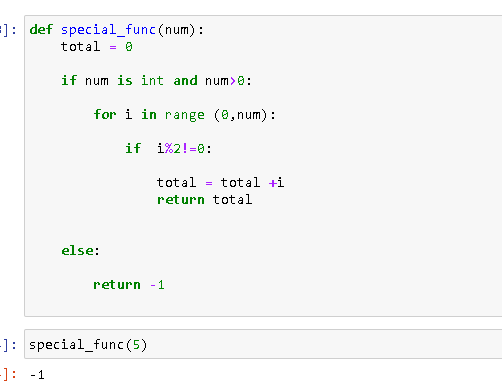For loop question no 88

def special_func(num):
total = 0
if type(num) is int and num > 0:
total = 1 # 1 is always a factor
i = 3 # because 2 will be a even facotor
while i <= num:
if num%i == 0 and i%2 != 0:
total = total + i
i = i + 1
else:
return -10

Why are you considering i =3??

Why are you dividing num%i??What is the problem with my code??